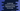# How to convert a comma-separated string to an array in JavaScript## How to convert a comma separated string to an array in JavaScript:

In this post, we will learn different ways to convert a comma separated string to an array. For example, we can convert the string one,two,three,four,five to the array [‘one’, ‘two’, ‘three’, ‘four’, ‘five’]. This is a common problem we faced in frontend or backend development. JavaScript provides a couple of functions those can be used to do it easily.

Let me show you these functions with examples:

### split():

We can pass ’,’ to the split function as its parameter and it will return the words in the string in an array. If you don’t provide any parameter to split, it breaks the string at blank spaces.

For example,

``````let givenString = 'one,two,three,four,five';

let arr = givenString.split(',');

console.log(arr);``````

It will split the string and put the words in the array arr. If you run this program, it will print the below output:

``[ 'one', 'two', 'three', 'four', 'five' ]``

### split and empty string:

If there is an empty string, i.e. two , without a word in between, it will put one empty string to the array. For example,

``````let givenString = 'one,two,,,,three';

let arr = givenString.split(',');

console.log(arr);``````

It will put three empty strings to the array.

``[ 'one', 'two', '', '', '', 'three' ]``

If you want to remove these empty strings, you can array a filter operation to the result array to remove all strings with 0 length.

``````let givenString = 'one,two,,,,three';

let arr = givenString.split(',').filter(e => e && e.length > 0);

console.log(arr);``````

Or, you can do this:

``````let givenString = 'one,two,,,,three';

let arr = givenString.split(',').filter(e => e);

console.log(arr);``````

We can also have strings with leading and trailing spaces and you might want to remove these spaces before doing any other processing. For example,

``````let givenString = 'one  ,two   ,,,,   three';

let arr = givenString.split(',').filter(e => e);

console.log(arr);``````

It will print:

``[ 'one  ', 'two   ', '   three' ]``

So, if we want to remove the leading and trailing spaces from these words, we can iterate through the array items using map and remove the spaces using trim():

``````let givenString = 'one  ,two   ,,,,   three';

let arr = givenString.split(',').filter(e => e).map(e => e.trim());

console.log(arr);``````

It will print:

``[ 'one', 'two', 'three' ]``

### split and regular expression/regex:

We can pass a regular expression or regex to split. For example, in the below example program,

``````let givenString = 'one  ,two   ,,,,   three';

let arr = givenString.split(/\s*,+\s*/);

console.log(arr);``````

This regular expression will remove all leading and trailing spaces from the words and also it will also remove the empty strings. Basically, this regular expression will remove the use of filter and map.

So, if you run this code, it will give the below result:

``[ 'one', 'two', 'three' ]``